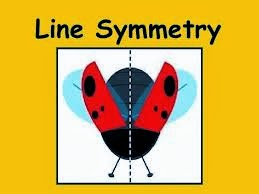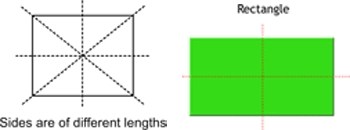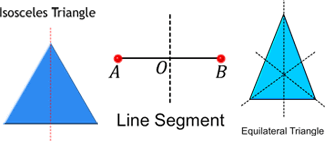## 24 January 2014

The word symmetry comes from the Greek word symmetroswhich means even.
A figure has line symmetry if a line can be drawn dividing it into two identical parts. The line is called the line of symmetry or axis of symmetry.Line symmetry is also known as reflection symmetry because a mirror line resembles the line of symmetry, where one half is the mirror image of the other half. Remember, while looking at a mirror, an object placed on the right appears to be on the left, and vice versa.
• For a line segment, the perpendicular bisector is the line of symmetry.
• For an equilateral triangle, the bisectors of the internal angles are the lines of symmetry.
• For a square, the lines of symmetry are the diagonals and the lines joining the mid-points of the opposite sides.
• The lines of symmetry of a rectangle are the lines joining the mid-points of the opposite sides.
• The line of symmetry of an isosceles triangle is the perpendicular bisector of the non-equal side.
• scalene triangle, in which all the sides are of different lengths, doesn’t have any line of symmetry.#### Regular polygon:

• polygon is said to be a regular polygon if all its sides are equal in length and all its angles are equal in measure. If a polygon is not a regular polygon, then it is said to be an irregular polygon.
• Regular and irregular polygons have lines of symmetry. An equilateral triangle is regular because each of its sides has the same length, and each of its angles measures sixty degrees.
• The number of lines of symmetry in a regular polygon is equal to the number of sides that it has.
• pentagon has five lines of symmetry.
• Similarly, a regular octagon has eight sides, and therefore, it will have eight lines of symmetry, while a regular decagonhas ten sides, so it will have ten lines of symmetry.

#### Irregular polygon:

• Most irregular polygons do not have line symmetry. However, some of them do. Look at the rectangle and the isosceles triangle. A rectangle has two lines of symmetry, and an isosceles triangle has one line of symmetry.
• Some letters have line symmetry.
• The letters A, B, C, D, E, I, K, M, T, U, V, W and Y have one line of symmetry.
• The letter H overlaps perfectly both vertically and horizontally. So it has two lines of symmetry.
• Similarly, the letter X has two lines of symmetry.
• The letters F, G, J, L, N, P, Q, R, S and Z have no line of symmetry.

 With our free PDF notes you can get success in any competitive or entrance exams like CTET,  KVS, NET, CAT, MAT, CMAT, SSC, B.ED, IBPS Recruitment, IAS, CSAT, State Civil Services Exams, UPTET, PSTET, HTET & many more. It also provides NCERT solutions, CBSE, NTSE, Olympiad study material, Indian General Knowledge, English, Hindi, Mathematics, Current affairs, Science, S.ST, model test papers, important Questions and Answers asked in CBSE examinations.